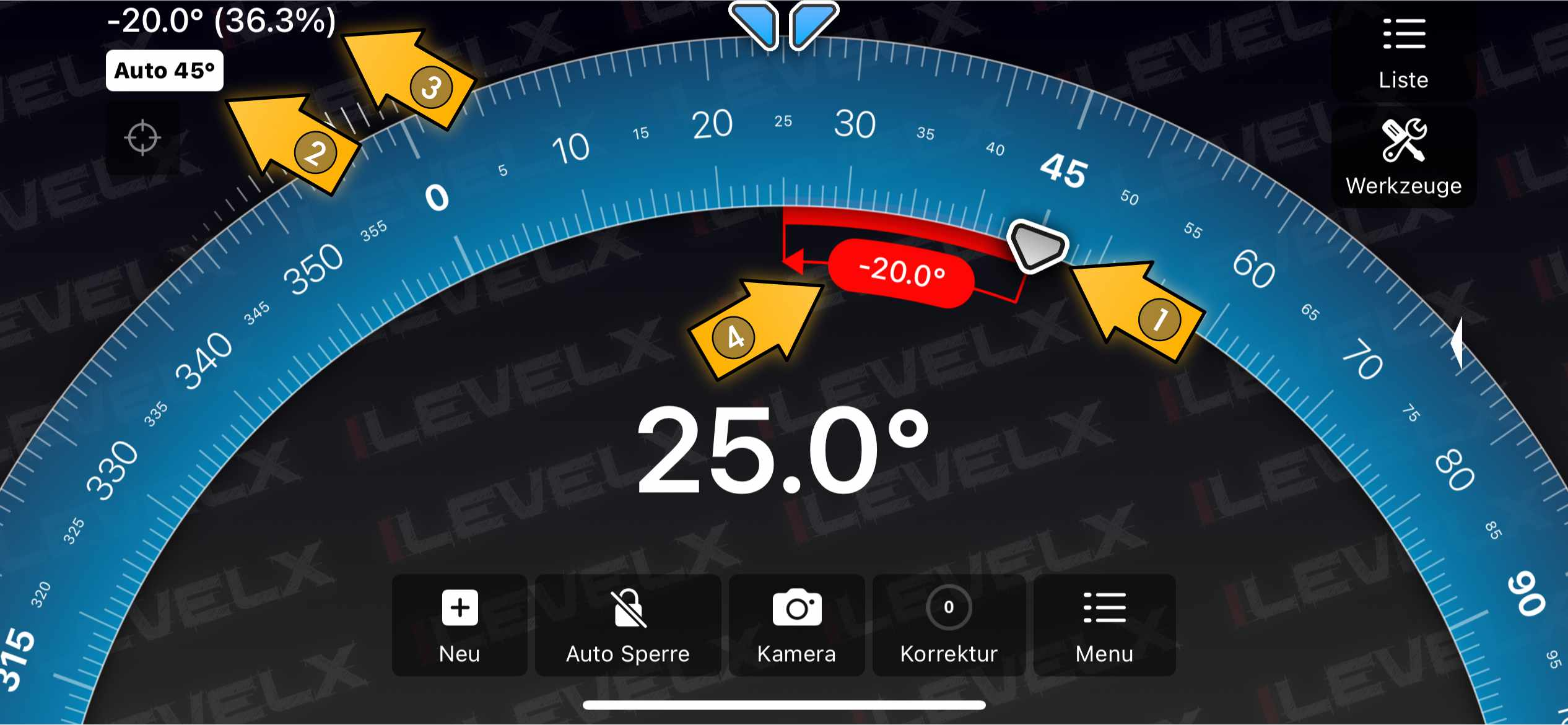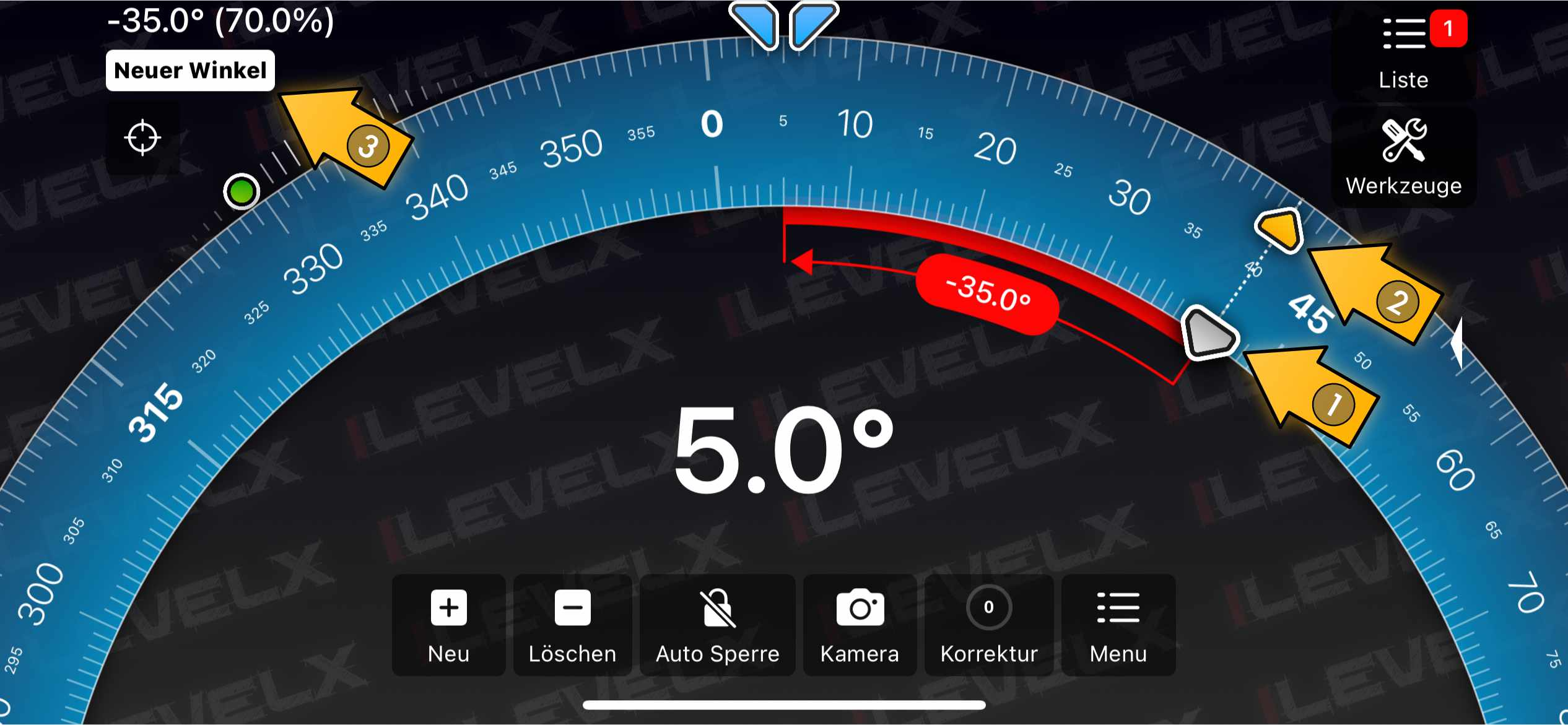## Understanding the reference angle

iLevel can easily be used to measure differences between angles. The difference is measured between the current (continuously measured) angle and a reference angle.

There a two types of reference angles:

1. An automatically selected nearest cardinal angle (0°, 45°, 90° and so on).
2. A stored angle from previous measurments.

### 1) Nearest cardinal angle

As long as there is no stored angle selected, iLevel will automatically set the nearest cardinal angle as the reference angle. This allows you to find deviations of edges from those commonly used angles. By this iLevel can be used as a spirit level/bubble level for all cardinal angles.

### 2) Stored angle

In iLevel you can store a virtually unlimited number of angular values (e.g. the slope of a roof beam) for future use. When selecting a stored angle, this angle will serve as the reference angle.

## Measuring difference to the nearest cardinal angle

The picture below show how to measure the difference to the nearest cardinal angle. The currently measured angle (25°) is 20° below the reference angle.

• Marker 1 indicates the reference angle marker (here it is automatically set to 45°).
• Marker 2 displays the reference name. Since it is automatically set, it’s labeled “AUTO 45°”.
• Marker 3 highlights the numeric angular difference.
• Marker 4 highlights the visualized angular difference.## Measuring difference to a stored angle

The picture below show how to measure the difference to a stored angle. The currently measured angle (5°) is 35° below the reference angle (40°).

• Marker 1 indicates the reference angle marker (here it is locked to the stored angle 45°).
• Marker 2 highlights the stored angle marker (yellow).
• Marker 3 highlights the name of the selected stored angle.